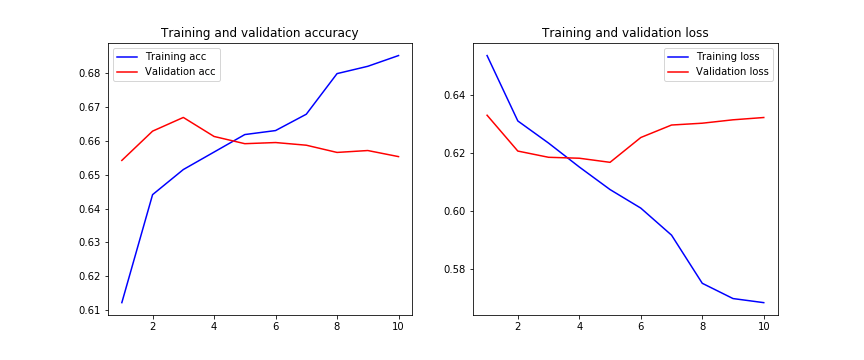# #StackBounty: #python #tensorflow #keras #deep-learning #cnn Text classification CNN overfits training

### Bounty: 50

I am trying to use a CNN architecture to classify text sentences. The architecture of the network is as follows:

``````text_input = Input(shape=X_train_vec.shape[1:], name = "Text_input")

conv2 = Conv1D(filters=128, kernel_size=5, activation='relu')(text_input)
drop21 = Dropout(0.5)(conv2)
pool1 = MaxPooling1D(pool_size=2)(drop21)
conv22 = Conv1D(filters=64, kernel_size=5, activation='relu')(pool1)
drop22 = Dropout(0.5)(conv22)
pool2 = MaxPooling1D(pool_size=2)(drop22)
dense = Dense(16, activation='relu')(pool2)

flat = Flatten()(dense)
dense = Dense(128, activation='relu')(flat)
out = Dense(32, activation='relu')(dense)

outputs = Dense(y_train.shape, activation='softmax')(out)

model = Model(inputs=text_input, outputs=outputs)
# compile
``````

I have some callbacks as early_stopping and reduceLR to stop the training and to reduce the learning rate when the validation loss is not improving (reducing).

``````early_stopping = EarlyStopping(monitor='val_loss',
patience=5)
model_checkpoint = ModelCheckpoint(filepath=checkpoint_filepath,
save_weights_only=False,
monitor='val_loss',
mode="auto",
save_best_only=True)
learning_rate_decay = ReduceLROnPlateau(monitor='val_loss',
factor=0.1,
patience=2,
verbose=1,
mode='auto',
min_delta=0.0001,
cooldown=0,
min_lr=0)
``````

Once the model is trained the history of the training goes as follows:We can observe here that the validation loss is not improving from epoch 5 on and that the training loss is being overfitted with each step.

I will like to know if I’m doing something wrong in the architecture of the CNN? Aren’t enough the dropout layers to avoid the overfitting? Which are other ways to reduce overfitting?

Any suggestion?

Edit:

I have tried also with regularization an the result where even worse:

``````kernel_regularizer=l2(0.01), bias_regularizer=l2(0.01)
``````Edit 2:

I have tried to apply BatchNormalization layers after each convolution and the result is the next one:

``````norm = BatchNormalization()(conv2)
``````

Get this bounty!!!

This site uses Akismet to reduce spam. Learn how your comment data is processed.